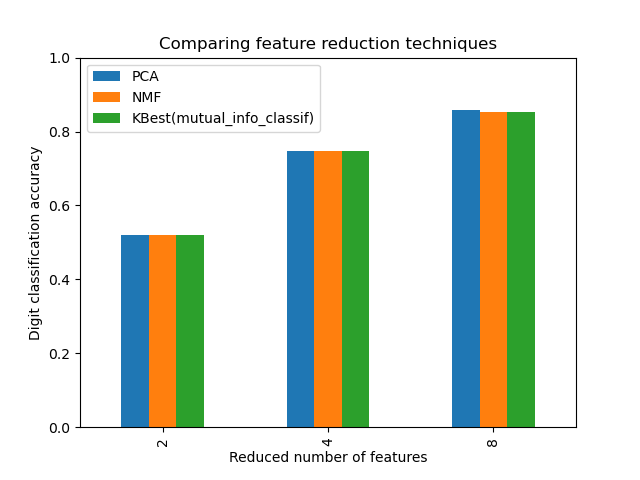# Selecting dimensionality reduction with Pipeline and GridSearchCV¶

This example constructs a pipeline that does dimensionality reduction followed by prediction with a support vector classifier. It demonstrates the use of GridSearchCV and Pipeline to optimize over different classes of estimators in a single CV run – unsupervised PCA and NMF dimensionality reductions are compared to univariate feature selection during the grid search.

Additionally, Pipeline can be instantiated with the memory argument to memoize the transformers within the pipeline, avoiding to fit again the same transformers over and over.

Note that the use of memory to enable caching becomes interesting when the fitting of a transformer is costly.

# Authors: Robert McGibbon
#          Joel Nothman
#          Guillaume Lemaitre


## Illustration of Pipeline and GridSearchCV¶

import numpy as np
import matplotlib.pyplot as plt
from sklearn.model_selection import GridSearchCV
from sklearn.pipeline import Pipeline
from sklearn.svm import LinearSVC
from sklearn.decomposition import PCA, NMF
from sklearn.feature_selection import SelectKBest, mutual_info_classif
from sklearn.preprocessing import MinMaxScaler

pipe = Pipeline(
[
("scaling", MinMaxScaler()),
# the reduce_dim stage is populated by the param_grid
("reduce_dim", "passthrough"),
("classify", LinearSVC(dual=False, max_iter=10000)),
]
)

N_FEATURES_OPTIONS = [2, 4, 8]
C_OPTIONS = [1, 10, 100, 1000]
param_grid = [
{
"reduce_dim": [PCA(iterated_power=7), NMF(max_iter=1_000)],
"reduce_dim__n_components": N_FEATURES_OPTIONS,
"classify__C": C_OPTIONS,
},
{
"reduce_dim": [SelectKBest(mutual_info_classif)],
"reduce_dim__k": N_FEATURES_OPTIONS,
"classify__C": C_OPTIONS,
},
]
reducer_labels = ["PCA", "NMF", "KBest(mutual_info_classif)"]

grid = GridSearchCV(pipe, n_jobs=1, param_grid=param_grid)
grid.fit(X, y)

GridSearchCV(estimator=Pipeline(steps=[('scaling', MinMaxScaler()),
('reduce_dim', 'passthrough'),
('classify',
LinearSVC(dual=False,
max_iter=10000))]),
n_jobs=1,
param_grid=[{'classify__C': [1, 10, 100, 1000],
'reduce_dim': [PCA(iterated_power=7, n_components=8),
NMF(max_iter=1000)],
'reduce_dim__n_components': [2, 4, 8]},
{'classify__C': [1, 10, 100, 1000],
'reduce_dim': [SelectKBest(score_func=<function mutual_info_classif at 0x7f0d7038c280>)],
'reduce_dim__k': [2, 4, 8]}])
In a Jupyter environment, please rerun this cell to show the HTML representation or trust the notebook.

import pandas as pd

mean_scores = np.array(grid.cv_results_["mean_test_score"])
# scores are in the order of param_grid iteration, which is alphabetical
mean_scores = mean_scores.reshape(len(C_OPTIONS), -1, len(N_FEATURES_OPTIONS))
# select score for best C
mean_scores = mean_scores.max(axis=0)
# create a dataframe to ease plotting
mean_scores = pd.DataFrame(
mean_scores.T, index=N_FEATURES_OPTIONS, columns=reducer_labels
)

ax = mean_scores.plot.bar()
ax.set_title("Comparing feature reduction techniques")
ax.set_xlabel("Reduced number of features")
ax.set_ylabel("Digit classification accuracy")
ax.set_ylim((0, 1))
ax.legend(loc="upper left")

plt.show()## Caching transformers within a Pipeline¶

It is sometimes worthwhile storing the state of a specific transformer since it could be used again. Using a pipeline in GridSearchCV triggers such situations. Therefore, we use the argument memory to enable caching.

Warning

Note that this example is, however, only an illustration since for this specific case fitting PCA is not necessarily slower than loading the cache. Hence, use the memory constructor parameter when the fitting of a transformer is costly.

from joblib import Memory
from shutil import rmtree

# Create a temporary folder to store the transformers of the pipeline
location = "cachedir"
memory = Memory(location=location, verbose=10)
cached_pipe = Pipeline(
[("reduce_dim", PCA()), ("classify", LinearSVC(dual=False, max_iter=10000))],
memory=memory,
)

# This time, a cached pipeline will be used within the grid search

# Delete the temporary cache before exiting
memory.clear(warn=False)
rmtree(location)


The PCA fitting is only computed at the evaluation of the first configuration of the C parameter of the LinearSVC classifier. The other configurations of C will trigger the loading of the cached PCA estimator data, leading to save processing time. Therefore, the use of caching the pipeline using memory is highly beneficial when fitting a transformer is costly.

Total running time of the script: ( 0 minutes 43.276 seconds)

Gallery generated by Sphinx-Gallery